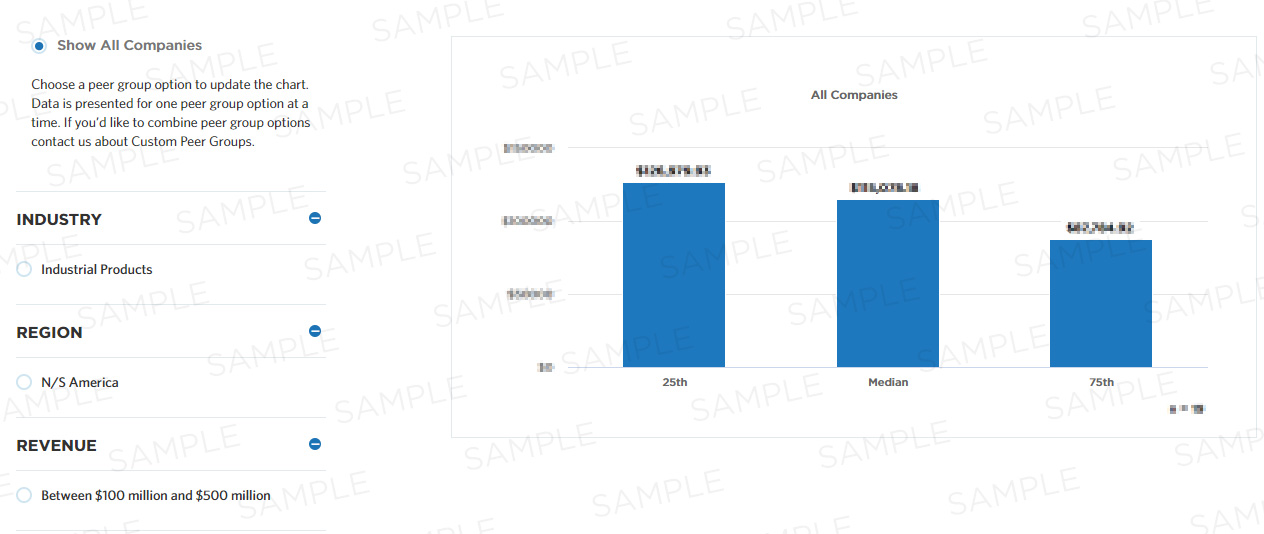# Percentage of total receipts that are processed error free the first time

This measure calculates the percentage of total receipts that are processed error free the first time. It is part of a set of Process Efficiency measures that help companies optimize their "process accounts receivable (AR)" process by minimizing waste and refining resource consumption.

## Benchmark Data

Measure Category:
Process Efficiency
Measure Id:
101758
Total Sample Size:
3,443 All Companies
Performers:
25th
Median
75th
Key Performance
Indicator:
Yes## Compute this Measure

Units for this measure are percent.

`(Number of receipts processed error-free first time / Number of receipts processed) * 100`

## Key Terms

#### Process efficiency

Process efficiency represents how well a process converts its inputs into outputs. A process that converts 100% of the inputs into outputs without waste is more efficient than one that converts a similar amount of inputs into fewer outputs.

#### Median

The metric value which represents the 50th percentile of a peer group. This could also be communicated as the metric value where half of the peer group sample shows lower performance than the expressed metric value or half of the peer group sample shows higher performance than the expressed metric value.